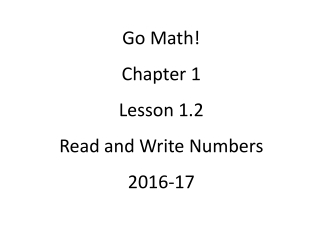DownloadDownload PresentationGo Math! Chapter 1 Lesson 1.2 Read and Write Numbers 2016-17

# Go Math! Chapter 1 Lesson 1.2 Read and Write Numbers 2016-17

Télécharger la présentation## Go Math! Chapter 1 Lesson 1.2 Read and Write Numbers 2016-17

- - - - - - - - - - - - - - - - - - - - - - - - - - - E N D - - - - - - - - - - - - - - - - - - - - - - - - - - -
##### Presentation Transcript

1. Go Math! Chapter 1 Lesson 1.2 Read and Write Numbers 2016-17

2. Place Value, Addition, and Subtraction

3. 816,537 30 10,000 500 7 800,000 6,000

4. In the number 83,995 the value of the 9 in the hundreds place is ten times the value of the 9 in the tens place.

5. The value of the 2 in 234 is ten times the value of the 3.

6. The value of the 4 in the thousands place in 4,143 is ten times the value of the 4 in the tens place.

7. Hundred Thousands Ten Thousands Thousands Hundreds Tens Ones X 10 X 10 X 10 X 10 X 10 X 100 X 1,000

8. The value of the 3 in 35,684 is 10 times greater than the value of the 3 in 143,692.

9. How can youread and write numbers through hundred thousands?

10. standard form 942,861

11. word form nine hundred forty-two thousand, eight hundred sixty-one

12. 65,983 381,394 502,103 316,040 86,200 941,008 12,802 600,005201,060 50,009 460,700 522,392 760,015 104,001 77,720 943,682 23,040 880,080 206,004 914,325 84,019

13. expanded form 900,000 + 40,000 + 2,000 + 800 + 60 + 1

14. Standard Form

15. 648,352 digits commas

16. Standard Form Expanded Form

17. 648,352 Standard Form 600,000 + sum + + 8,000 40,000 values 300 + + 2 50

18. Standard Form Expanded Form Word Form

19. 648,352 Standard Form Expanded Form six hundred forty-eight words thousand, three hundred fifty-two

20. Ima Student

21. Forms of a Number Standard Form Expanded Form Word Form 4

22. Table of Contents Choosing the Operation 1 Problem Solving Steps 2 Place and Value 3 Forms of a Number 4

23. Let’s Practice!

24. Write the expanded form. 8,352 8,000 + 300 + 50 + 2

25. Write the expanded form. 7,865 7,000 + 800 + 60 + 5

26. Write the expanded form. 4,069 4,000 + 60 9 +

27. Write the expanded form. 35,613 30,000 + 5,000 + 600 + 10 3 +

28. Write the expanded form. 96,407 90,000 + 6,000 + 400 + 7

29. Write the expanded form. 472,108 400,000 + 70,000 + 2,000 + 100 8 +

30. Write the expanded form. 603,942 600,000 + 3,000 + 900 + 40 2 +

31. Write the standard form. 7,000 + 800 + 60 + 5 7,865

32. Write the standard form. 4,000 + 300 + 8 4,308

33. Write the standard form. 50,000 + 4,000 + 600 + 90 54,690

34. Write the standard form. 80,000 + 700 + 20 + 4 80,724

35. Write the standard form. 600,000 + 40,000 + 800 +30 + 9 640,839

36. Write the standard form. 90,000 + 3,000 + 60 + 2 93,062

37. Write the standard form. 500,000 + 7,000 + 20 507,020

38. Write the standard form. two thousand, three hundred fifty-eight 2,358

39. Write the standard form. forty thousand, nine hundred twenty 40,920

40. Write the standard form. five thousand, eight hundred six 5,806

41. Write the standard form. fifteen thousand, two hundred fourteen 15,214

42. Write the standard form. sixty-one thousand, thirty-two 61,032

43. Write the standard form. eight hundred forty-five thousand, two hundred twenty-one 845,221

44. Write the standard form. six hundred forty thousand, three hundred seventy 640,370

45. Write the standard form. seven hundred one thousand, four 701,004

46. Write the standard form. two hundred thousand, sixty 200,060

47. Write the word form. 86,234 eighty-six thousand, two hundred thirty-four

48. Write the word form. 75,092 seventy-five thousand, ninety-two

49. Write the word form. 400,005 four hundred thousand, five